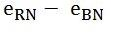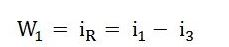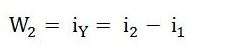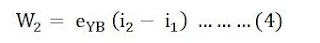# Two Wattmeter Method of Power Measurement

0
1039

### Measurement of power by the two wattmeter method in star connection:

#### In this method, we use two-watt meters and the current coils of these watt meters are connected in series with two lines and potential coils are connected across these two lines and the load is connected in the star as shown in the following figure.#### The voltage across the potential coil of watt meter W1 is#### Instantaneous power measured by the wattmeter, W1 is given by,#### The voltage across the potential coil of watt meter W2 is#### Instantaneous power measured by the wattmeter, W2 is given by#### Adding equations 1 and 2 we get following results.### Measurement of Power by the two wattmeter method in delta connection:

#### In this method also we use two-watt meters and the current coils of these watt meters are connected in series with two lines and potential coils are connected across these two lines and the load is connected in delta as shown in the following figure.#### The instantaneous current flowing through the watt meter W1 is,#### Instantaneous power measured by the wattmeter, W1 is given by,#### The instantaneous current flowing through the watt meter W2 is,#### Instantaneous power measured by the wattmeter, W2 is given by,#### The total 3 phase power will be the sum of power measured by watt meters W1 and W2